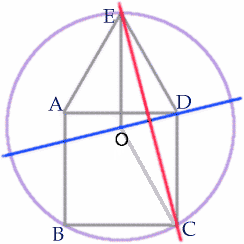#### You may also like### Doodles

Draw a 'doodle' - a closed intersecting curve drawn without taking pencil from paper. What can you prove about the intersections?### Russian Cubes

I want some cubes painted with three blue faces and three red faces. How many different cubes can be painted like that?### Picture Story

Can you see how this picture illustrates the formula for the sum of the first six cube numbers?

# Encircling

##### Age 14 to 16Challenge Level

Congratulations James Page of Hethersett High School, Norwich on your solution to this question. Again there are many possible methods of showing that the radius of the circle is equal to the side of the square.The blue line $OD$ is a diameter of the circle and a line of symmetry.

$\angle EDC = 150^{\circ}$ and $\triangle ECD$ isosceles so $\angle CED = \angle CDE =15^{\circ}$.

Now $\angle OED = 30^{\circ}$ so $\angle OEC = \angle OED - \angle CED = 15^{\circ}$ and hence the red line $EC$ is a line of symmetry for the quadrilateral $OEDC$ proving that the radius of the circle, $OC$ is equal in length to the side of the square.